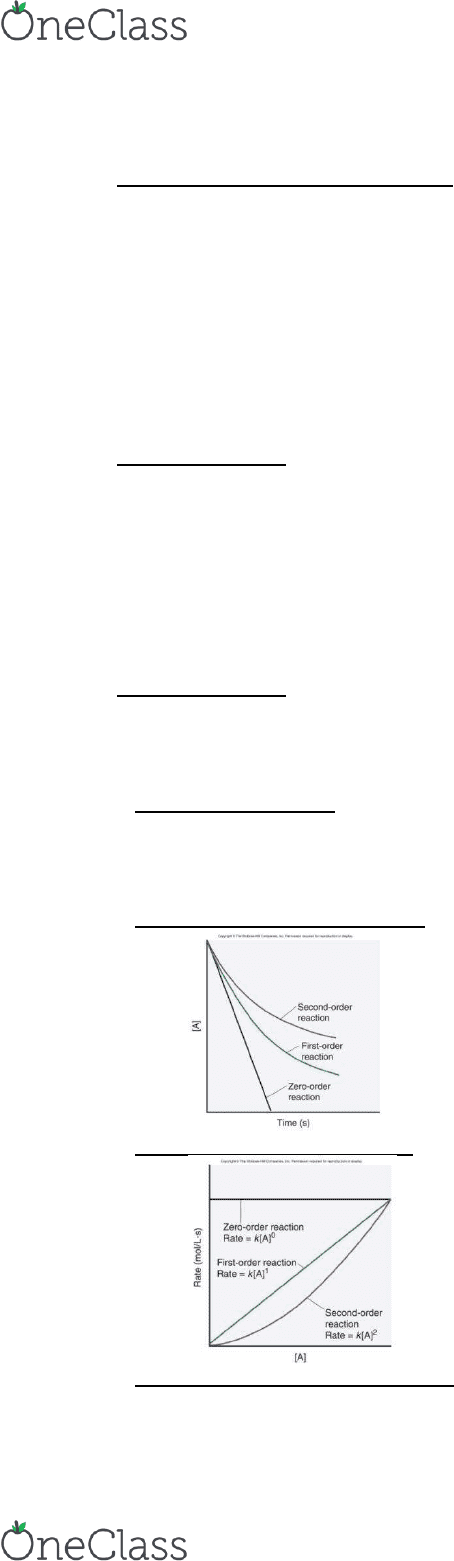Study Guides (380,000)
US (220,000)
CU-Boulder (1,000)
CHEM (40)
Midterm

# CHEM 1133 Midterm: Chapter 16 Chemical Kinetics Study Guide

Department
Chemistry
Course Code
CHEM 1133
Professor
Ruth Heisler
Study Guide
Midterm

This preview shows pages 1-2. to view the full 7 pages of the document.Chapter 16 Chemical Kinetics
Equilibrium vs. Thermodynamics vs. Kinetics
o Equilibrium constants help us predict how far a reaction will go (what it looks like)
o Thermodynamics allows us to predict the extent of a reaction, but it tells us nothing about the speed of the rxn or
how it occurs
o Kinetics is the study of the rates and mechanisms of chemical rxns (how do they get there)
H2+1/2O2 H20 E=-237 KJ
Favorable according to thermodynamics but no rxn is observed under normal conditions (too slow --
kinetics)
Factors that influence reaction rates
o Particles must collide in order to react
o The higher the concentration of reactants, the greater the rxn rate
A higher concentration of reactant particles allows a greater number of collisions
o The physical state of the reactants influences rxn rate
Substances must mix in order for particles to collide -- increased surface area to interact
o The higher the temperature, the greater the rxn rate
At a higher temp, particles have more energy and can collide more often and more effectively
Expressing the Reaction Rate
o Reaction rate is measured in terms of the changes in concentrations of reactants of products per unit of time
o      
     


o Square brackets indicate a concentration in moles per liter
Rates of chemical reactions
o The rate is a measure of how fast a reaction occurs
o The rates are proportional to the stoichiometry of the reaction
o The reaction rate is defined as the change in concentration of reactants or products per unit of time
o Consider a generic rxn: reactant products
The rate of disappearance is measured by the change in concentration of one of the reactants divided by
the change in time
   

The rate of formation is measured by the change in concentration of one of the products divided by the
change in time
    

Units are M/s
o General rxn: aA + bB cC + dD
Rate of reaction = rate of disappearance of reactant
OR
Rate of reaction = rate of formation of product
  

  
  

By convention, the rxn rate is always a positive number
o Solving for rates:
Average rate: use the rate equation above 
   

Always positive
Rate of change of a specific reactant or product: just use   

Can be negative or positive
Average vs. Instantaneous Rates
o Reaction rates typically change during the course of the reaction
Faster at the beginning and then slow as equilibrium is approached
o The average rate of the reaction is the change in concentration divided by the change in time
o The instantaneous rate of the reaction is the |slope| of the tangent line drawn at the time of interest
The instantaneous rate at t=0 is called the initial rate
The slope and rate decrease over time as equilibrium is approached
This rate is the important rate of reaction
The rate law
o For any general reaction occurring at a fixed temp: aA + bB cC + dD
  
find more resources at oneclass.com
find more resources at oneclass.com

Only pages 1-2 are available for preview. Some parts have been intentionally blurred.o The term k is the rate constant, which is specific for a given reaction at a given temperature
o The exponents m and n are reaction orders and are determined by experiment
The values of m and n are not necessarily related in any way to the coefficients a and b
Effect on concentration of reaction rate
o The rate of reaction often depends on the concentration of one or more of the reactants
The rate law shows the relationship between the rate of the reaction and concentration of the reactant
A products
Rate=k[A]n
k= rate constant
n=reaction order
The value of n is usually an integer and determines how the rate depends on the concentration of the
reactant
n=0 - zero order - rate is independent of [A]
N=1 - first order - rate is proportional to [A]
N=2 - second order - rate is proportional to [A]2
Zero order reaction
o [A] decreases linearly with time
o Rate = k[A]0 = k
o Rate is independent of [A]
o Slope is constant
Rate is constant
o K has units of M s-1
o Zero order reactions occur under conditions where the amount of reactant actually available for a reaction is
unaffected by changes in the overall quantity of the reactant
Ex: sublimation (solid to gas)
First order reaction
o Rate= k[A]
o Rate is directly proportional to [A]
o Rate slows down as the reaction proceeds
o K has units of s-1
Second Order Reaction
o Rate= k[A]2
o Rate is proportional to [A]2
Rate is more sensitive to [A]
o K has units of M-1 s-1
Reactant Concentrations vs. Time
o
Rate vs. Reactant Concentration
o
Reaction order for multiple reactants
o When reactions contain 2 or more reactants, the order is specified with respect to each reactant and the overall
order
o Rate laws for any reaction can only be determined from experiments
find more resources at oneclass.com
find more resources at oneclass.com
###### You're Reading a Preview

Unlock to view full version